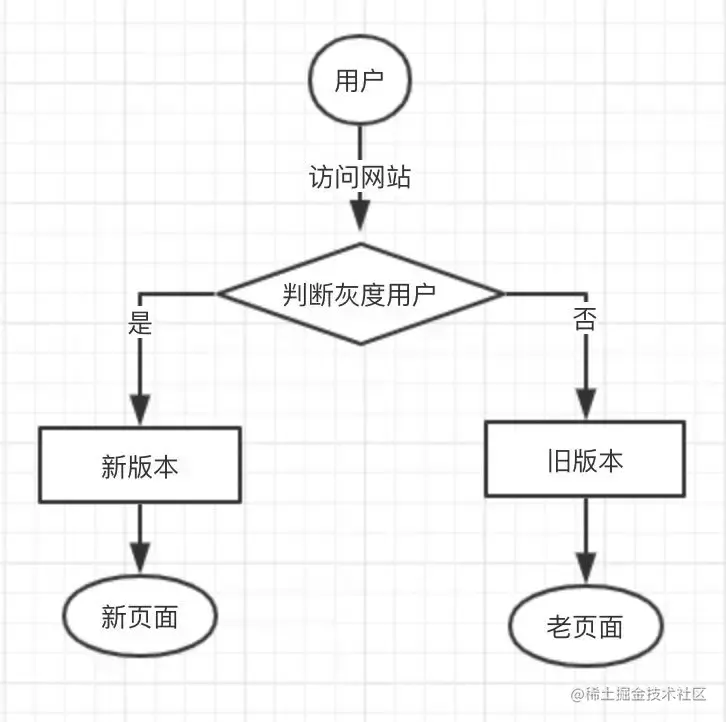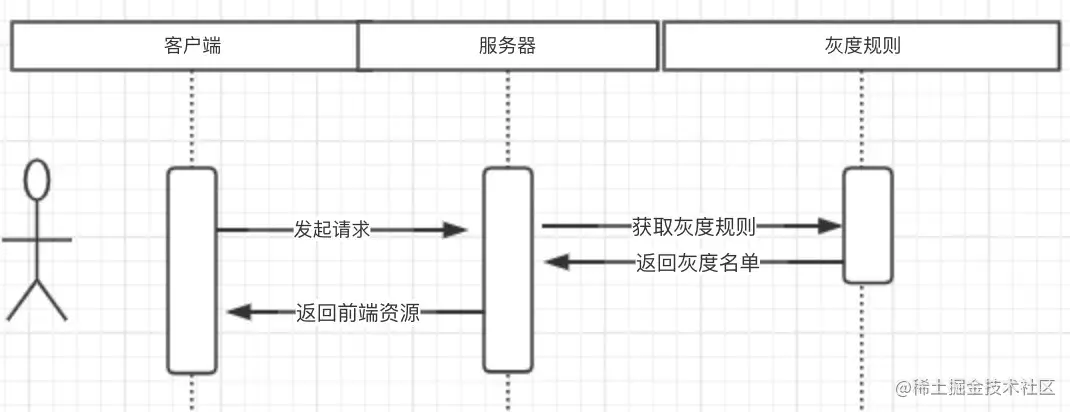# 要进大厂？前端灰度发布必须要知道

### 一. 灰度发布是什么？### 二.灰度发布的优点

1.提前收集用户使用意见，及时完善产品功能
2.控制未知异常只出现在小范围内，不影响大多数用户
3.发现产品是否存在外在问题（如合规），可及时回滚至已旧版本

1.放量规则

2.资源新旧版本

### 四. 灰度实现原理### 五. 前端灰度发布的几种方式

1.服务端渲染应用``````// 服务端代码
// 静态模板
const model1 = () => {
return `
<!DOCTYPE html>
<html>
<meta charset="utf-8">
<meta http-equiv="X-UA-Compatible" content="IE=edge">
<body>
<div id="mydiv">我是A界面</div>
</body>
</html>
`
}
const model2 = () => {
return `
<!DOCTYPE html>
<html>
<meta charset="utf-8">
<meta http-equiv="X-UA-Compatible" content="IE=edge">
<body>
<div id="mydiv">我是B界面</div>
</body>
</html>
`
}
const isPass = getRule(req.uid) // 查询规则
if (isPass) { // 在白名单
res.render(model2)
} else {
res.render(model1)
}
...复制代码``````

2.前后端半分离的应用

``````<!DOCTYPE html>
<html>
<meta charset="utf-8">
<meta http-equiv="X-UA-Compatible" content="IE=edge">
<body>
<div id="react-content"></div>
</body>
<script src="http://cdn.com/1.0.1/my.js"></script>
</html>复制代码``````

``````// 服务端代码
const isPass = getRule(req.uid) // 查询规则
let version = 1.0.1; // 旧版本号
if (isPass) { // 在白名单
version = 2.0.0
}
// 静态模板
const model = version => {
return `
<!DOCTYPE html>
<html>
<meta charset="utf-8">
<meta http-equiv="X-UA-Compatible" content="IE=edge">
<body>
<div id="react-content"></div>
</body>
<script src="http://cdn.com/{version}/my.js"></script>
</html>
`
}
res.render(model(version))  // 返回带版本号的模板复制代码``````

3. 客户端渲染的前端应用

``````// 客户端端代码
// 组件
const component1 = () => {
return (<div>我是A组件</div>)
}
const component2 = () => {
return (<div>我是B组件</div>)
}
const isPass = \$.ajax('/getRule?uid') // 查询规则
...
render() {
if (isPass) { // 在白名单
return model2()
} else {
return model1()
}
}
...复制代码``````

``````// 客户端端代码
const syncLoadJs = function (version, fn) {
const oScript = document.createElement('script');
oScript.setAttribute('src',`https://cdn.com/{version}/my.js`);
oScript.setAttribute('type','text/javascript');
oScript.onerror = function() { window.location.href = '/error.htm' };
document.body.appendChild(oScript);
},

...
const isPass = \$.ajax('/getRule?uid') // 查询规则
let version = 1.0.1; // 旧版本号
if (isPass) { // 在白名单
version = 2.0.0
}